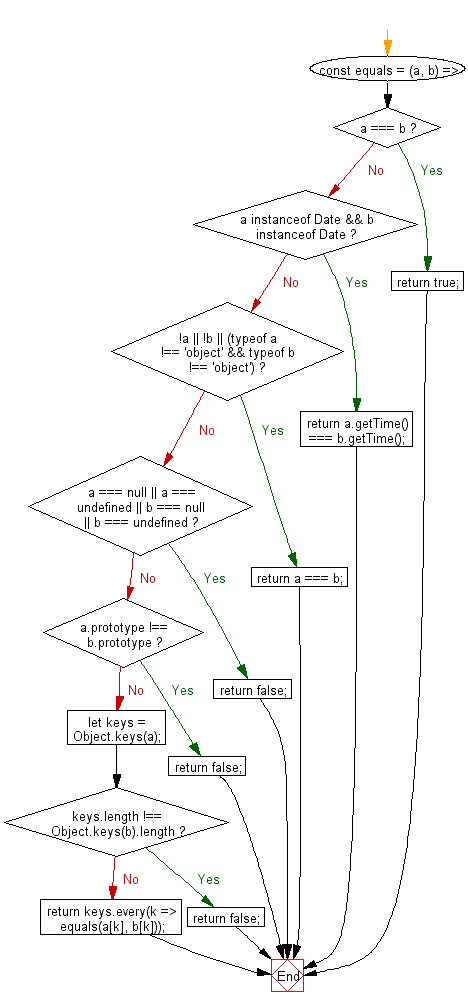# JavaScript: Perform a deep comparison between two values to determine if they are equivalent

## JavaScript fundamental (ES6 Syntax): Exercise-47 with Solution

Write a JavaScript program to perform a deep comparison between two values to determine if they are equivalent.

Note: Check if the two values are identical, if they are both Date objects with the same time, using Date.getTime() or if they are both non-object values with an equivalent value (strict comparison). Check if only one value is null or undefined or if their prototypes differ. If none of the above conditions are met, use Object.keys() to check if both values have the same number of keys, then use Array.every() to check if every key in the first value exists in the second one and if they are equivalent by calling this method recursively.

• Check if the two values are identical, if they are both Date objects with the same time, using Date.prototype.getTime() or if they are both non-object values with an equivalent value (strict comparison).
• Check if only one value is null or undefined or if their prototypes differ.
• If none of the above conditions are met, use Object.keys() to check if both values have the same number of keys.
• Use Array.prototype.every() to check if every key in a exists in b and if they are equivalent by calling equals() recursively.

Sample Solution:

JavaScript Code:

``````//#Source https://bit.ly/2neWfJ2
const equals = (a, b) => {
if (a === b) return true;
if (a instanceof Date && b instanceof Date) return a.getTime() === b.getTime();
if (!a || !b || (typeof a !== 'object' && typeof b !== 'object')) return a === b;
if (a === null || a === undefined || b === null || b === undefined) return false;
if (a.prototype !== b.prototype) return false;
let keys = Object.keys(a);
if (keys.length !== Object.keys(b).length) return false;
return keys.every(k => equals(a[k], b[k]));
};

console.log(equals({ a: [2, { e: 3 }], b: , c: 'foo' }, { a: [2, { e: 3 }], b: , c: 'foo' }));
```
```

Sample Output:

```true
```

Flowchart:Live Demo:

See the Pen javascript-basic-exercise-1-47 by w3resource (@w3resource) on CodePen.

Improve this sample solution and post your code through Disqus

What is the difficulty level of this exercise?

Test your Programming skills with w3resource's quiz.

﻿

## JavaScript: Tips of the Day

Chunks an array into n smaller arrays

Example:

```const tips_chunkIntoN = (arr, n) => {
const size = Math.ceil(arr.length / n);
return Array.from({ length: n }, (v, i) =>
arr.slice(i * size, i * size + size)
);
}
console.log(tips_chunkIntoN([1, 2, 3, 4, 5, 6, 7,8], 4));
```

Output:

```[[1,2],[3,4],[5,6],[7,8]]
```